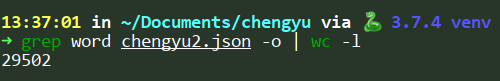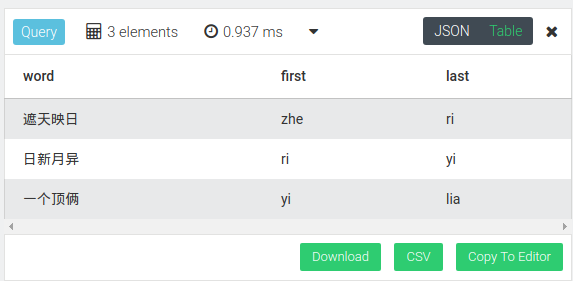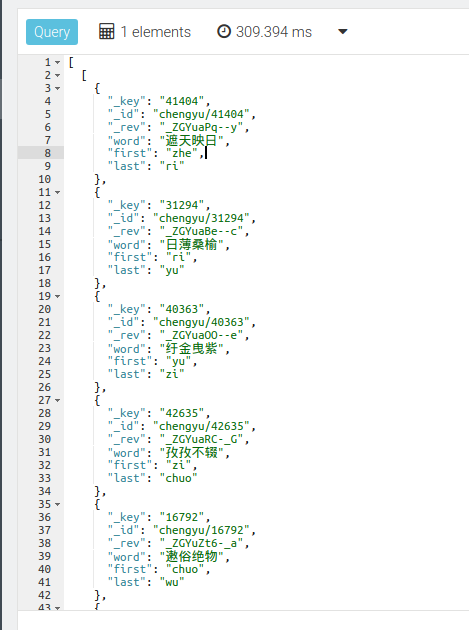# 实验过程## 插入成语到数据库里

def import_chengyu():
file = open("chengyu2.json", "r")

coll = chengyu_db.collection("chengyu")

chengyu_regular = []

for cy in chengyu:
chengyu_regular.append({
"word": cy["word"],
"first": cy["pinyin_first"],
"last": cy["pinyin_last"]
})

coll.insert_many(chengyu_regular)


## 构建图的边

chengyu_cur = chengyu_db.aql.execute("FOR c IN chengyu RETURN c")
chengyu = []

chengyu_map = {}

for cy in tqdm(chengyu_cur, unit_scale=True):
first = cy["first"]
chengyu.append(cy)

if chengyu_map.get(first):
chengyu_map[first].append(cy)
else:
chengyu_map[first] = [cy]



edges = []
for cy in tqdm(chengyu, unit_scale=True):
last = cy["last"]

nexts = chengyu_map.get(last)

if not nexts:
continue

for n in nexts:
edges.append({
"_from": "chengyu/{}".format(cy["_key"]),
"_to": "chengyu/{}".format(n["_key"])
})


edges_chunks = []

for i in range(0, len(edges), 2000):
edges_chunks.append(edges[i: i + 2000])

for edges_chunk in tqdm(edges_chunks, unit_scale=True):
edges_coll.insert_many(edges_chunk)


## 查询

for c in chengyu
filter c.word == "一个顶俩"
return c


for c in chengyu
filter c.word == "遮天映日"
return c


for v in OUTBOUND
SHORTEST_PATH "chengyu/41404" to "chengyu/43795" chengyu_edge
return {word: v.word, first: v.first, last: v.last}for v,e,p in 10 outbound "chengyu/41404" chengyu_edge
filter v._id == "chengyu/43795"
limit 1
return p.vertices